The SEVERITY Procedure

Example 23.3 Defining a Model for Mixed-Tail Distributions

In some applications, a few severity values tend to be extreme as compared to the typical values. The extreme values represent the worst case scenarios and cannot be discarded as outliers. Instead, their distribution must be modeled to prepare for their occurrences. In such cases, it is often useful to fit one distribution to the non-extreme values and another distribution to the extreme values. The mixed-tail distribution mixes two distributions: one for the body region, which contains the non-extreme values, and another for the tail region, which contains the extreme values. The tail distribution is usually a generalized Pareto distribution (GPD), because it is usually good for modeling the conditional excess severity above a threshold. The body distribution can be any distribution. The following definitions are used in describing a generic formulation of a mixed-tail distribution: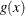PDF of the body distribution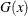CDF of the body distribution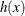PDF of the tail distribution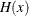CDF of the tail distributionscale parameter for the body distribution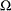set of nonscale parameters for the body distribution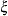shape parameter for the GPD tail distribution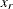normalized value of the response variable at which the tail starts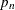mixing probability

Given these notations, the PDF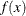and the CDF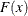of the mixed-tail distribution are defined as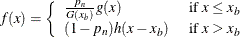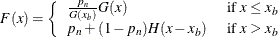where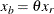is the value of the response variable at which the tail starts.

These definitions indicate the following:

• The body distribution is conditional on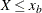, where X denotes the random response variable.

• The tail distribution is the generalized Pareto distribution of the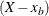values.

• The probability that a response variable value belongs to the body is. Consequently the probability that the value belongs to the tail is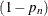.

The parameters of this distribution are,,,, and. The scale of the GPD tail distribution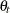is computed as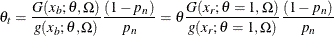The parameteris usually estimated using a tail index estimation algorithm. One such algorithm is the Hill’s algorithm (Danielsson et al., 2001), which is implemented by the predefined utility function SVRTUTIL_HILLCUTOFF available to you in the Sashelp.Svrtdist library. The algorithm and the utility function are described in detail in the section Predefined Utility Functions. The function computes an estimate of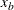, which can be used to compute an estimate ofbecause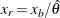, where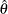is the estimate of the scale parameter of the body distribution.

The parameteris usually determined by the domain expert based on the fraction of losses that are expected to belong to the tail.

The following SAS statements define the LOGNGPD distribution model for a mixed-tail distribution with the lognormal distribution as the body distribution and GPD as the tail distribution:

/*------- Define Lognormal Body-GPD Tail Mixed Distribution -------*/
proc fcmp library=sashelp.svrtdist outlib=work.sevexmpl.models;
function LOGNGPD_DESCRIPTION() $256; length desc$256;
desc1 = "Lognormal Body-GPD Tail Distribution.";
desc2 = " Mu, Sigma, and Xi are free parameters.";
desc3 = " Xr and Pn are constant parameters.";
desc = desc1 || desc2 || desc3;
return(desc);
endsub;

function LOGNGPD_SCALETRANSFORM() $3; length xform$3;
xform = "LOG";
return (xform);
endsub;

subroutine LOGNGPD_CONSTANTPARM(Xr,Pn);
endsub;

function LOGNGPD_PDF(x, Mu,Sigma,Xi,Xr,Pn);
cutoff = exp(Mu) * Xr;
p = CDF('LOGN',cutoff, Mu, Sigma);
if (x < cutoff + constant('MACEPS')) then do;
return ((Pn/p)*PDF('LOGN', x, Mu, Sigma));
end;
else do;
gpd_scale = p*((1-Pn)/Pn)/PDF('LOGN', cutoff, Mu, Sigma);
h = (1+Xi*(x-cutoff)/gpd_scale)**(-1-(1/Xi))/gpd_scale;
return ((1-Pn)*h);
end;
endsub;

function LOGNGPD_CDF(x, Mu,Sigma,Xi,Xr,Pn);
cutoff = exp(Mu) * Xr;
p = CDF('LOGN',cutoff, Mu, Sigma);
if (x < cutoff + constant('MACEPS')) then do;
return ((Pn/p)*CDF('LOGN', x, Mu, Sigma));
end;
else do;
gpd_scale = p*((1-Pn)/Pn)/PDF('LOGN', cutoff, Mu, Sigma);
H = 1 - (1 + Xi*((x-cutoff)/gpd_scale))**(-1/Xi);
return (Pn + (1-Pn)*H);
end;
endsub;

subroutine LOGNGPD_PARMINIT(dim,x[*],nx[*],F[*],Ftype,
Mu,Sigma,Xi,Xr,Pn);
outargs Mu,Sigma,Xi,Xr,Pn;
array xe / nosymbols;
array nxe / nosymbols;

eps = constant('MACEPS');

Pn = 0.8; /* Set mixing probability */
_status_ = .;
call streaminit(56789);
Xb = svrtutil_hillcutoff(dim, x, 100, 25, _status_);
if (missing(_status_) or _status_ = 1) then
Xb = svrtutil_percentile(Pn, dim, x, F, Ftype);

/* prepare arrays for excess values */
i = 1;
do while (i <= dim and x[i] < Xb+eps);
i = i + 1;
end;
dime = dim-i+1;
call dynamic_array(xe, dime);
call dynamic_array(nxe, dime);
j = 1;
do while(i <= dim);
xe[j] = x[i] - Xb;
nxe[j] = nx[i];
i = i + 1;
j = j + 1;
end;

/* Initialize lognormal parameters */
call logn_parminit(dim, x, nx, F, Ftype, Mu, Sigma);
if (not(missing(Mu))) then
Xr = Xb/exp(Mu);
else
Xr = .;

/* Initialize GPD's shape parameter using excess values */
call gpd_parminit(dime, xe, nxe, F, Ftype, theta_gpd, Xi);
endsub;

subroutine LOGNGPD_LOWERBOUNDS(Mu,Sigma,Xi,Xr,Pn);
outargs Mu,Sigma,Xi,Xr,Pn;

Mu   = .; /* Mu has no lower bound */
Sigma = 0; /* Sigma > 0 */
Xi   = 0; /* Xi > 0 */
endsub;
quit;



Note the following points about the LOGNGPD definition:

• The parametersandare not estimated with the maximum likelihood method used by PROC SEVERITY, so you need to specify them as constant parameters by defining the dist_CONSTANTPARM subroutine. The signature of LOGNGPD_CONSTANTPARM subroutine lists only the constant parameters Xr and Pn.

• The parameteris estimated by first using the SVRTUTIL_HILLCUTOFF utility function to compute an estimate of the cutoff point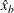and then computing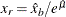. If SVRTUTIL_HILLCUTOFF fails to compute a valid estimate, then the SVRTUTIL_PERCENTILE utility function is used to setto theth percentile of the data. The parameteris fixed to 0.8.

• The Sashelp.Svrtdist library is specified with the LIBRARY= option in the PROC FCMP statement to enable the LOGNGPD_PARMINIT subroutine to use the predefined utility functions (SVRTUTIL_HILLCUTOFF and SVRTUTIL_PERCENTILE) and parameter initialization subroutines (LOGN_PARMINIT and GPD_PARMINIT).

• The LOGNGPD_LOWERBOUNDS subroutine defines the lower bounds for all parameters. This subroutine is required because the parameter Mu has a non-default lower bound. The bounds for Sigma and Xi must be specified. If they are not specified, they are returned as missing values, which PROC SEVERITY interprets as having no lower bound. You need not specify any bounds for the constant parameters Xr and Pn, because they are not subject to optimization.

The following DATA step statements simulate a sample from a mixed-tail distribution with a lognormal body and GPD tail. The parameteris fixed to 0.8, the same value used in the LOGNGPD_PARMINIT subroutine defined previously.

/*----- Simulate a sample for the mixed-tail distribution -----*/
data testmixdist(keep=y label='Lognormal Body-GPD Tail Sample');
call streaminit(45678);
label y='Response Variable';
N = 100;
Mu = 1.5;
Sigma = 0.25;
Xi = 1.5;
Pn = 0.8;

/* Generate data comprising the lognormal body */
Nbody = N*Pn;
do i=1 to Nbody;
y = exp(Mu) * rand('LOGNORMAL')**Sigma;
output;
end;

/* Generate data comprising the GPD tail */
cutoff = quantile('LOGNORMAL', Pn, Mu, Sigma);
gpd_scale = (1-Pn) / pdf('LOGNORMAL', cutoff, Mu, Sigma);
do i=Nbody+1 to N;
y = cutoff + ((1-rand('UNIFORM'))**(-Xi) - 1)*gpd_scale/Xi;
output;
end;
run;



The following statements use PROC SEVERITY to fit the LOGNGPD distribution model to the simulated sample. They also fit three other predefined distributions (BURR, LOGN, and GPD). The final parameter estimates are written to the Work.Parmest data set.

/*--- Set the search path for functions defined with PROC FCMP ---*/
options cmplib=(work.sevexmpl);

/*-------- Fit LOGNGPD model with PROC SEVERITY --------*/
proc severity data=testmixdist print=all plots(histogram kernel)=all
outest=parmest;
loss y;
dist logngpd burr logn gpd;
run;



Some of the results prepared by PROC SEVERITY are shown in Output 23.3.1 through Output 23.3.4. The "Model Selection" table in Output 23.3.1 indicates that all models converged. The last table in Output 23.3.1 shows that the model with LOGNGPD distribution has the best fit according to almost all the statistics of fit. The Burr distribution model is the closest contender to the LOGNGPD model, but the GPD distribution model fits the data very poorly.

Output 23.3.1: Summary of Fitting Mixed-Tail Distribution

The SEVERITY Procedure

Input Data Set
Name WORK.TESTMIXDIST
Label Lognormal Body-GPD Tail Sample

Model Selection
Distribution Converged -2 Log
Likelihood
Selected
logngpd Yes 418.78232 Yes
Burr Yes 424.93728 No
Logn Yes 459.43471 No
Gpd Yes 558.13444 No

All Fit Statistics
Distribution -2 Log
Likelihood
AIC AICC BIC KS AD CvM
logngpd 418.78232 * 428.78232 * 429.42062 * 441.80817   0.62140 * 0.31670 * 0.04972 *
Burr 424.93728   430.93728   431.18728   438.75280 * 0.71373   0.57649   0.07860
Logn 459.43471   463.43471   463.55842   468.64505   1.55267   3.27122   0.48448
Gpd 558.13444   562.13444   562.25815   567.34478   3.43470   16.74156   3.31860

The plots in Output 23.3.2 show that both the lognormal and GPD distributions fit the data poorly, GPD being the worst. The Burr distribution fits the data as well as the LOGNGPD distribution in the body region, but has a poorer fit in the tail region than the LOGNGPD distribution.

The P-P plots of Output 23.3.3 provide a better visual confirmation that the LOGNGPD distribution fits the tail region better than the Burr distribution.

Output 23.3.2: Comparison of the CDF and PDF Estimates of the Fitted Models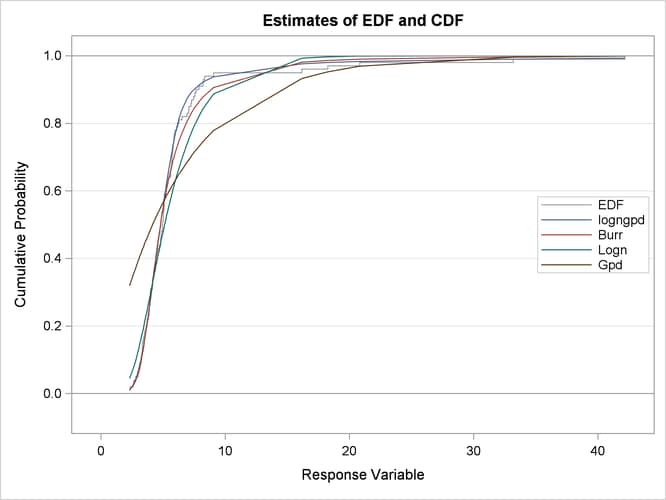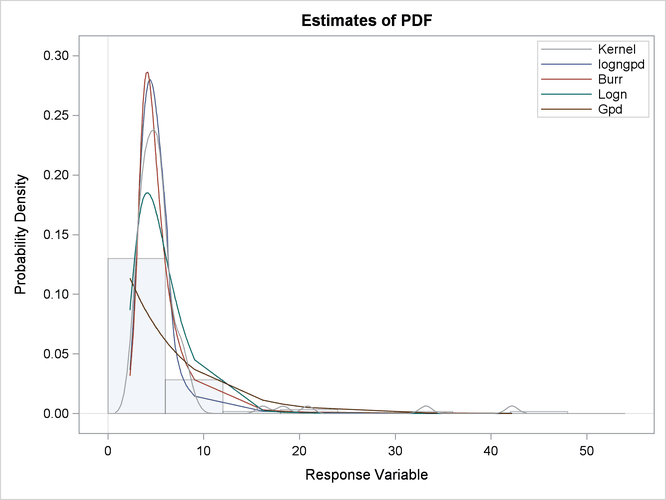Output 23.3.3: P-P Plots for the LOGNGPD and BURR Distribution Models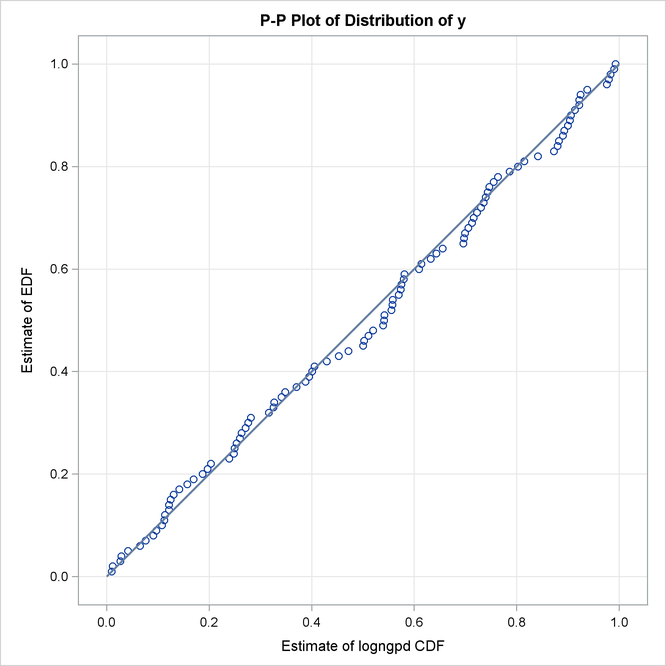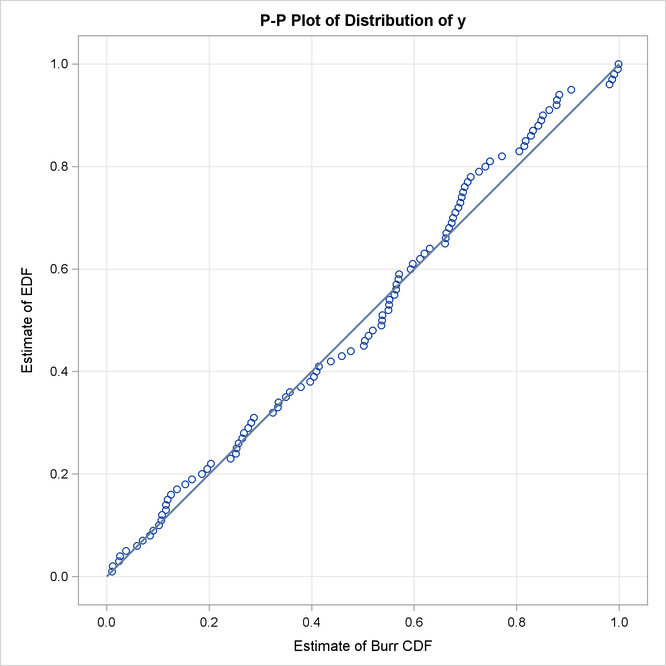The detailed results for the LOGNGPD distribution are shown in Output 23.3.4. The initial values table indicates the values computed by LOGNGPD_PARMINIT subroutine for the Xr and Pn parameters. It also uses the bounds columns to indicate the constant parameters. The last table in the figure shows the final parameter estimates. The estimates of all free parameters are significantly different from 0. As expected, the final estimates of the constant parameters Xr and Pn have not changed from their initial values.

Output 23.3.4: Detailed Results for the LOGNGPD Distribution

The SEVERITY Procedure
logngpd Distribution

Distribution Information
Name logngpd
Description Lognormal Body-GPD Tail Distribution. Mu, Sigma, and Xi are free parameters. Xr and Pn are constant parameters.
Distribution Parameters 5

Initial Parameter Values and Bounds
Parameter Initial
Value
Lower
Bound
Upper
Bound
Mu 1.49954 -Infty Infty
Sigma 0.76306 1.05367E-8 Infty
Xi 0.36661 1.05367E-8 Infty
Xr 1.27395 Constant Constant
Pn 0.80000 Constant Constant

Convergence Status
Convergence criterion (GCONV=1E-8) satisfied.

Optimization Summary
Optimization Technique Trust Region
Iterations 11
Function Calls 33
Failed Function Calls 1
Log Likelihood -209.39116

Parameter Estimates
Parameter Estimate Standard
Error
t Value Approx
Pr > |t|
Mu 1.57921 0.06426 24.57 <.0001
Sigma 0.31868 0.04459 7.15 <.0001
Xi 1.03771 0.38205 2.72 0.0078
Xr 1.27395 Constant . .
Pn 0.80000 Constant . .

The following SAS statements use the parameter estimates to compute the value where the tail region is estimated to start (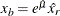) and the scale of the GPD tail distribution (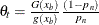):

/*-------- Compute tail cutoff and tail distribution's scale --------*/
data xb_thetat(keep=x_b theta_t);
set parmest(where=(_MODEL_='logngpd' and _TYPE_='EST'));
x_b = exp(Mu) * Xr;
theta_t = (CDF('LOGN',x_b,Mu,Sigma)/PDF('LOGN',x_b,Mu,Sigma)) *
((1-Pn)/Pn);
run;

proc print data=xb_thetat noobs;
run;



Output 23.3.5: Start of the Tail and Scale of the GPD Tail Distribution

x_b theta_t
6.18005 1.27865

The computed values ofandare shown as x_b and theta_t in Output 23.3.5. Equipped with this additional derived information, you can now interpret the results of fitting the mixed-tail distribution as follows:

• The tail starts at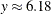. The primary benefit of using the scale-normalized cutoff () as the constant parameter instead of using the actual cutoff () is that the absolute cutoff gets optimized by virtue of optimizing the scale of the body region (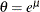).

• The values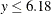follow the lognormal distribution with parameters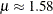and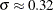. These parameter estimates are reasonably close to the parameters used for simulating the sample.

• The values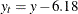(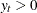) follow the GPD distribution with scale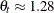and shape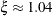.